# The function f(x) = - - may be represented by the power series 1-x Part 1:...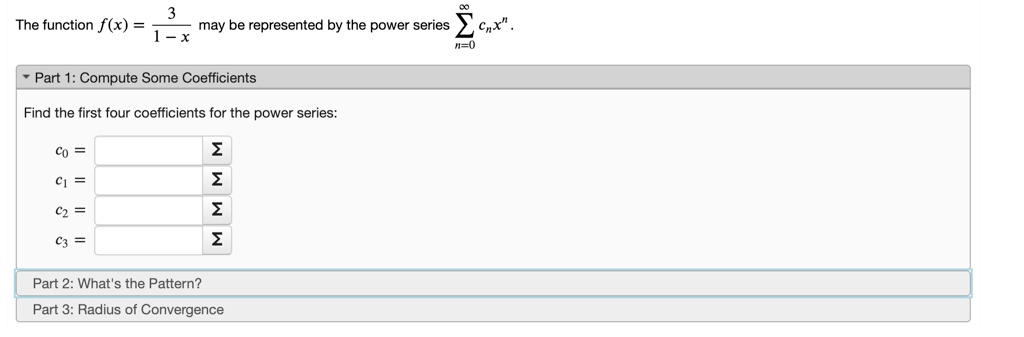The function f(x) = - - may be represented by the power series 1-x Part 1: Compute Some Coefficients Find the first four coefficients for the power series: MMMM Part 2: What's the Pattern? Part 3: Radius of Convergence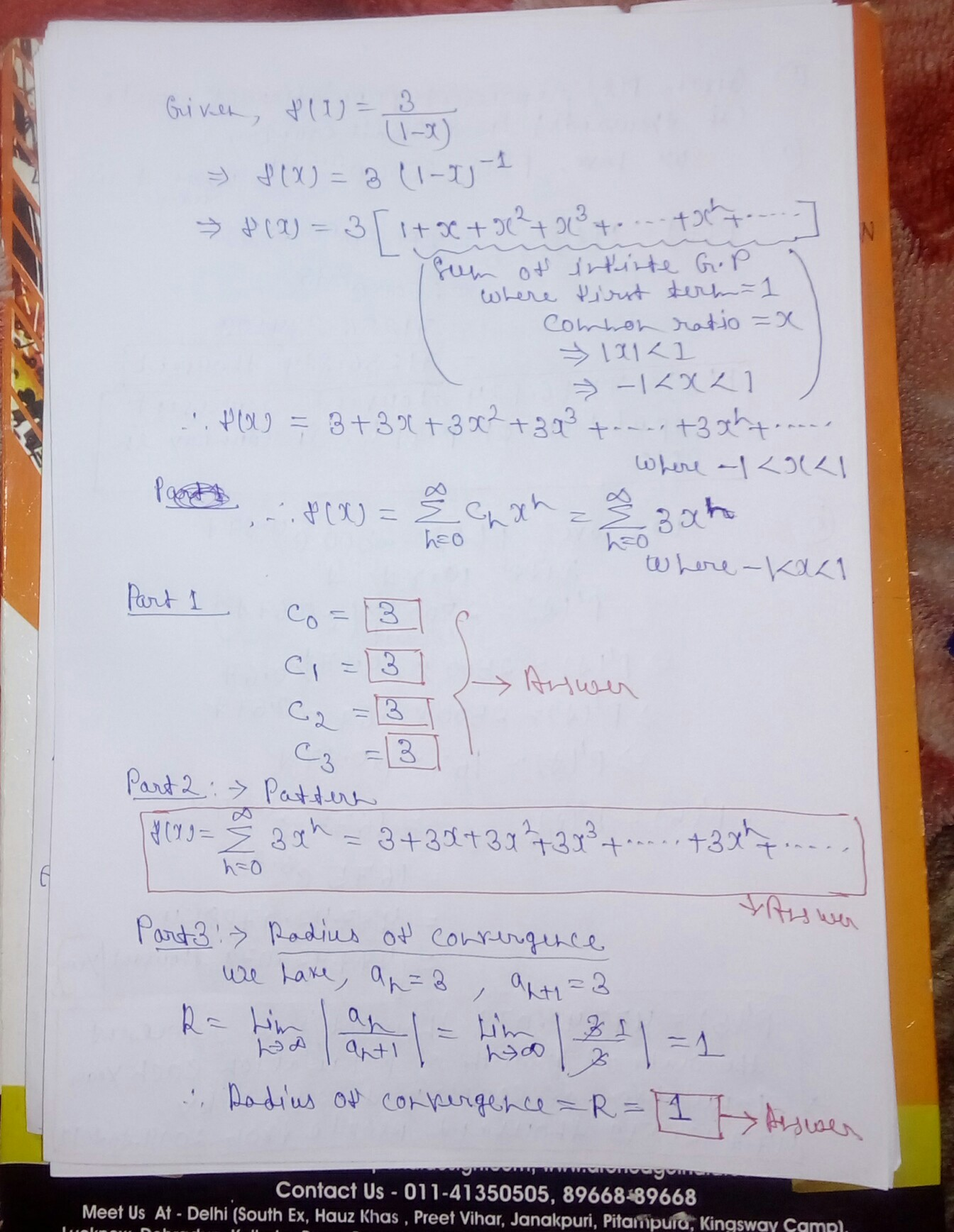#### Earn Coin

Coins can be redeemed for fabulous gifts.

Similar Homework Help Questions
• ### 3 is represented as a power series: (1 point) The function f(x) 1+36x2 Σ f(x) -...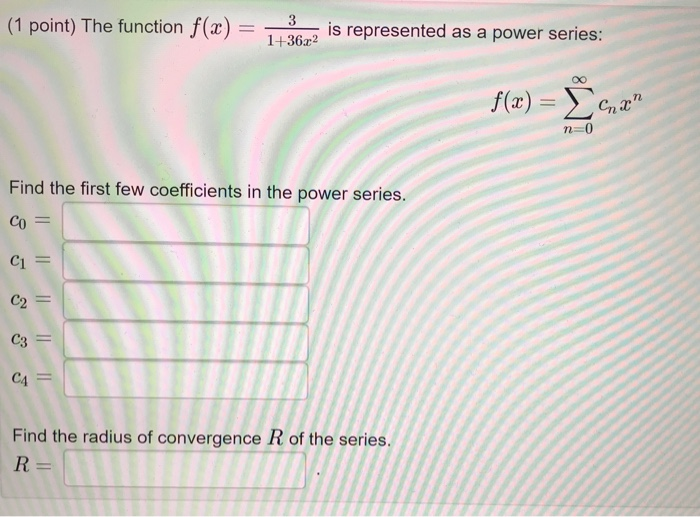3 is represented as a power series: (1 point) The function f(x) 1+36x2 Σ f(x) - n-0 Find the first few coefficients in the power series. CO CI C2 C3 CA Find the radius of convergence R of the series R =

• ### - (1 point) The function f(x) 4 (1-2x)2 is represented as a power series f(x) =...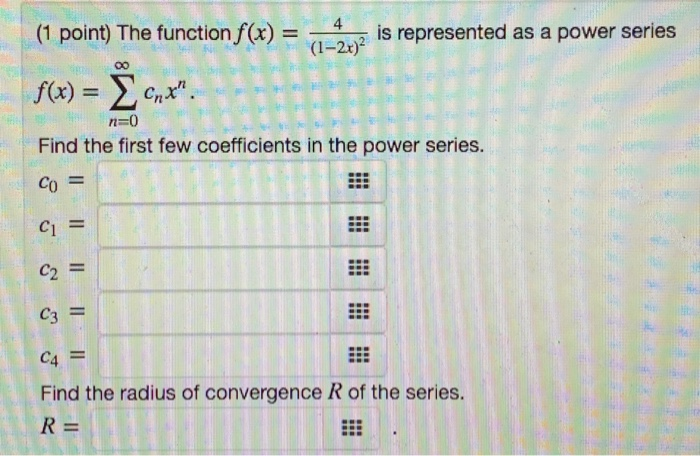- (1 point) The function f(x) 4 (1-2x)2 is represented as a power series f(x) = 0,*". n=0 Find the first few coefficients in the power series. Co = C1 = C2 = C3 = C4 = Find the radius of convergence R of the series. R=

• ### (1 point) The function f(x) = 7 (152) is represented as a power series 00 f(x)...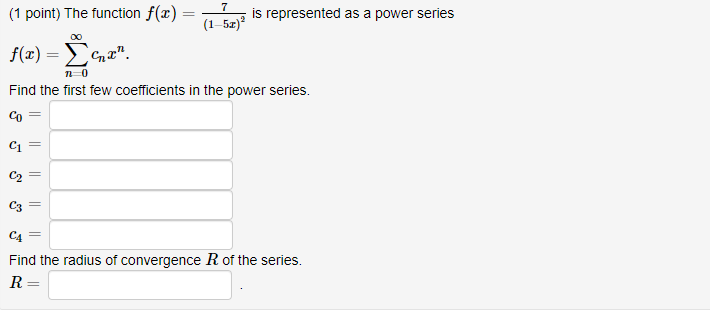(1 point) The function f(x) = 7 (152) is represented as a power series 00 f(x) = 42" 10 Find the first few coefficients in the power series. = C1 C2 = C3 C4 = Find the radius of convergence R of the series. R=

• ### 9 (1 point) The function f(1) = 11622 is represented as a power series: f(x) =...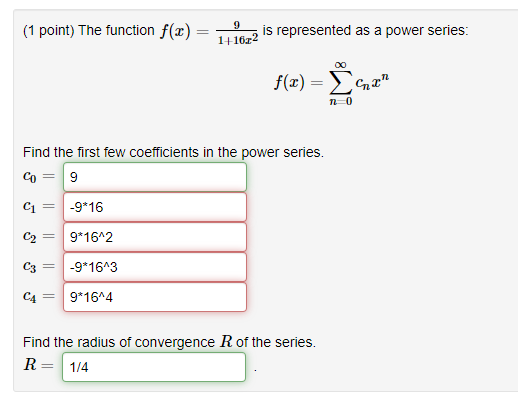9 (1 point) The function f(1) = 11622 is represented as a power series: f(x) = 42" Find the first few coefficients in the power series. Co = 9 C1 = -9*16 C2 = 9*16^2 C3 = -9*1643 9*16^4 Find the radius of convergence R of the series. R= 1/4

• ### (1 point) The function f(x) = 4x arctan(6x) is represented as a power series f(x) =...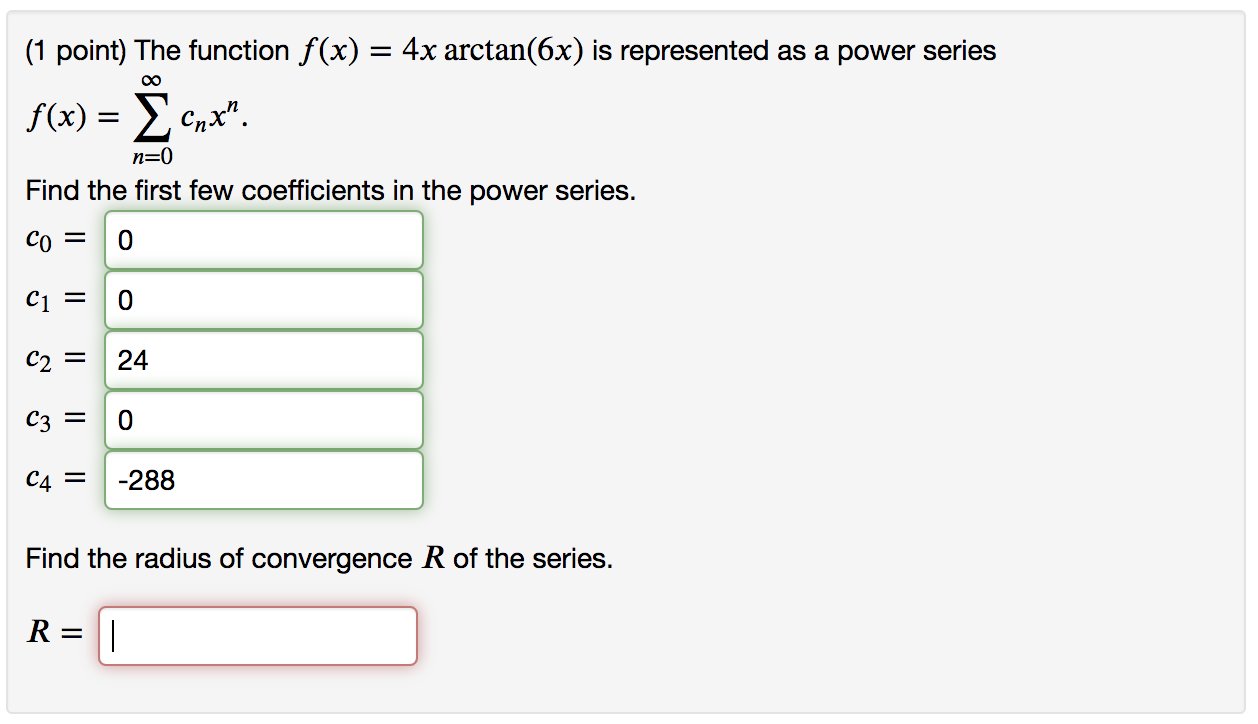(1 point) The function f(x) = 4x arctan(6x) is represented as a power series f(x) = Xcnx". n=0 Find the first few coefficients in the power series. co = 0 Ci = 0 C2 = 24 C3 = 0 C4 = -288 Find the radius of convergence R of the series. R= II

• ### (1 point) The function f(3) = ln(1 – z?) is represented as a power series f(3)...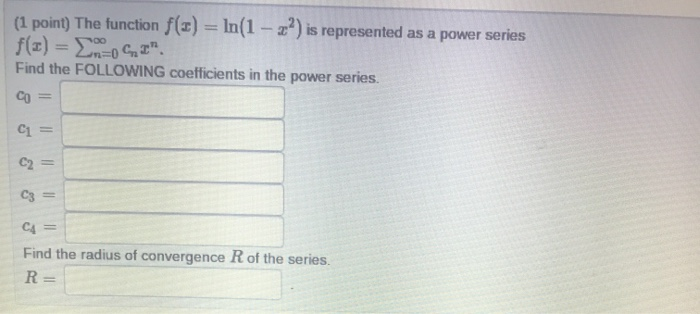(1 point) The function f(3) = ln(1 – z?) is represented as a power series f(3) = EMOCI" Find the FOLLOWING coefficients in the power series. Со Il C1 = C2 = C3 = C4 Find the radius of convergence R of the series. R=

• ### (1 point) The function f(x) = 6 (1 - 2x) can be represented as a power...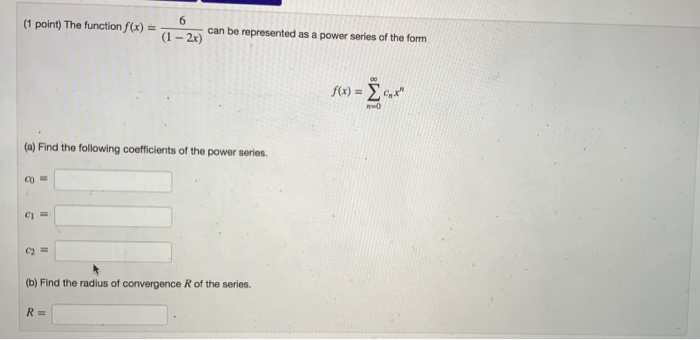(1 point) The function f(x) = 6 (1 - 2x) can be represented as a power series of the form f(x) = 6X" (a) Find the following coefficients of the power series. CO CU (b) Find the radius of convergence of the series. R=

• ### Express the function as a power series, find the first 5 coefficients of the terms, and...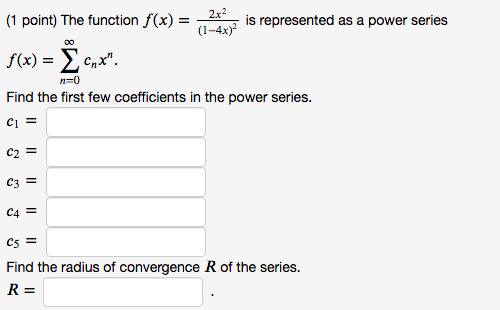Express the function as a power series, find the first 5 coefficients of the terms, and find the radius of convergence. (1 point) The function f(x) = 2x2 is represented as a power series (1-4x) f(x) = Xcnx". Find the first few coefficients in the power series. n=0 Ci = C2 = C3 = C4 = C5 = Find the radius of convergence R of the series. R=

• ### (1 pt) The function f(x) = 2x2 arctan (x*) is represented as a power series What is the lowest term with a nonzero coefficient. Find the radius of convergence R of the series. (1 pt) The functio...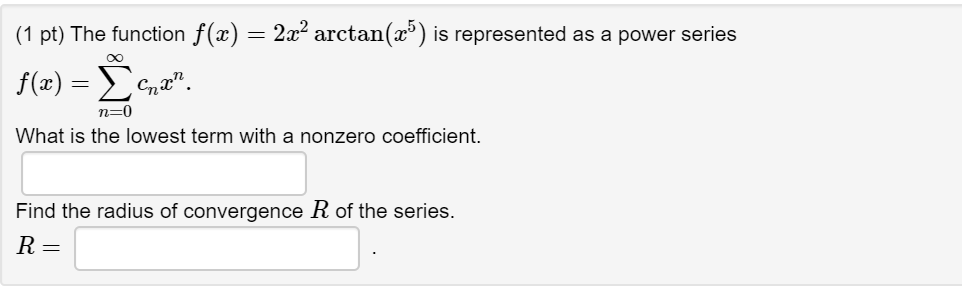(1 pt) The function f(x) = 2x2 arctan (x*) is represented as a power series What is the lowest term with a nonzero coefficient. Find the radius of convergence R of the series. (1 pt) The function f(x) = 2x2 arctan (x*) is represented as a power series What is the lowest term with a nonzero coefficient. Find the radius of convergence R of the series.

• ### 1. Represent the function 10/1−10x as a power series f(x)=∞∑n=0cn x^n Compute the first few coefficients...

1. Represent the function 10/1−10x as a power series f(x)=∞∑n=0cn x^n Compute the first few coefficients of this power series: c0=    c1=    c2=    c3=    c4= Find the radius of convergence R= 2. The Taylor series for f(x)=e^x at a = 2 is ∞∑n=0 cn(x−2)^n. Find the first few coefficients. c0=    c1=    c2= c3= c4=